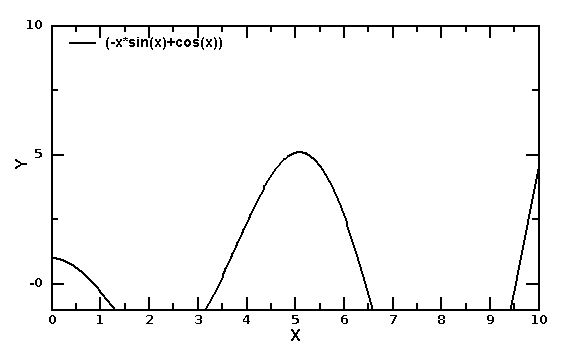# DMelt:Programming/7 Matlab and Octave

Limitted access. First login to DataMelt if you are a full DataMelt member. Then login to HandWiki as a user.

# Using Matlab/Octave

DataMelt can be used to program in MatLab/Octave programming language implemented in Java. For this purpose, DataMelt uses JMathLab engine to run execute the code. Unlike other scripting languages, such as Jython, Groovy or JRuby, JMathLab scripts are less interactive and, generally, are not integrated into the JVM. However, JMathLab scripts are mainly designed for symbolic calculations.

Here is a simple script which can be used in DataMelt:

syms x % symbolic x
y=diff(x*cos(x),x) %  differentiate x*cos(x)
plot2d('minx=0;maxx=10;miny=-1;maxy=10')  % make canvas
draw2d(y) % plot the result

Save this cript into a file, say "example.m" (note the extension *.m), and run this script as usual (click on the [run]) button. The result is a pop-up window with the image as shown below:Use JMathLab Shell for interactive programming, or the editor to create scripts and run them.

Look at the section DMelt:JMathlab/1_Introduction for more detail.